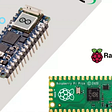# Virtual Machine Concept in Assembly Languages:

Computer Hardware and Software are related to each other through a concept called `Virtual Machine`. To Understand this concept consider a program written in High level language such as C++ or java.Now we already know that Computer executes instructions in machine code, so to convert this high level code into machine code we can use interpretation (line by line conversion to machine code) or Compilation of the entire program to machine code. In terms of virtual machine we can say that first the program gets is converted from high level code to assembly code by Virtual Machine 1 and then that Assembly is converted to Machine code by Virtual Machine 2.

# level 1:

level 1 can be the Digital Logic Hardware.

# level 2:

`Instruction Set Architecture` comes in level 2 where machine language is executed by a program embedded in computer microprocessor called microprogram.

# level 3:

This is where the `Assembly Language` operates which uses mnemonics such as ADD,SUB,MOV which are translated to machine language.

# level 4:

This is where most of us work today i.e. High Level Languages operate here which get translated by their respective compilers.

# Binary Integers:

A computer simply uses `0s` and `1s` at the lowest level representing `off` and `on` state. Bits are numbered sequentially starting at zero on the right side and increasing toward the left. The bit on the left is called the `most significant bit (MSB)`, and the bit on the right is the `least significant bit (LSB)`.

Things to remember about `Binary Integers`:

• Binary Integers can be signed or unsigned
• Signed Integers can be either positive or negative
• An unsigned integer is by default positive
• Zero is considered positive<
• Dots can be inserted after every four binary integers for readability

# Translating Unsigned Binary Integers to Decimal:

To translate Binary to Decimal we start from the left and multiply the each digit a power of `2`. for-example consider the binary number `110` will be equal to `6`. Solution: `2E2 x 1 + 2E1 x 1+ 2E0 x 0 = 6` where `2En` means 2 to the `raised power`.

# Translating Unsigned Decimals Integers to Binary:

To translate from Decimal to Binary we repeatedly divide the number by 2 saving each remainder as a binary digit.

We can concatenate the binary bits from the remainder column of the table in reverse order `(D 5 , D 4 , . . .)` to produce binary `100101`. Because computer storage always consists of binary numbers whose lengths are multiples of `8`, we fill the remaining two digit positions on the left with zeros, producing `00100101`.

When Adding Binary Number keep in mind that `1+1` will produce `10`with `1` being the carry to the next digit.

`00000100 + 00000111 = 00001011`

# Integer Storage Sizes (Data types):

Basic Storage unit in a `x86` computer is `byte` containing `8 bits`.Some of the data types are

• Byte 8bit
• Word 16bit
• Double Word 32bit

Hexadecimal Addition works the same way as decimal addition.Hexadecimal addition is used to locate new addresses in the memory. For example, let’s add the hexadecimal values 6A2 and 49A. In the lowest digit position,`2 + A = decimal 12`, so there is no carry and we use `C` to indicate the hexadecimal sumdigit. In the next position, `A + 9 = decimal 19`, so there is a carry because `19 ≥ 16` , the number base. We calculate `19 MOD 16 = 3`, and carry a 1 into the third digit position. Finally,we add `1 + 6 + 4 = decimal 11`, which is shown as the letter `B` in the third position of the sum. The hexadecimal sum is `B3C`.

So , `6A2 + 49A = B3C`

# Signed Binary Integers

Signed Binary Integers can be `positive` or `negative`.The `Most Significant Bit MSB` represents the sign: `0 is positive and 1 is negative`.

# Two’s-Complement Representation

`Negative Integers` use Two's-Complement Representation using the rule that two's complement of a number is its `additive inverse`. for-example consider a value 11111110, its two's complement will be:

Starting value00000001Step 1: Reverse the bits11111110Step 2: Add 1 to the value from Step 111111110 +00000001Sum: Two’s-complement representation11111111

In two’s complement of hexadecimal numbers we firstly reverse the number and then add 1 to it.To reverse the number we can subtract the number from `15`. Here are the examples:

`6A3D --> 95C2 + 1 --> 95C3` `95C3 --> 6A3C + 1 --> 6A3D`

# Converting Signed Binary to Decimal:

if the `Most Significant Bit` is `1` then it means the number is a `negative signed integer`.For example, signed binary `11110000` has a `1` in the highest bit, indicating that it is a negative integer.

Starting value 11110000

• Step 1: Reverse the bits 00001111
• Step 2: Add 1 to the value from above step 00001111 + 1
• Step 3: Create the two’s complement00010000
• Step 4: Convert to decimal =16

As the integer was negative so its answer is `-16`.

# Coverting Signed Decimal to Binary:

Following are the Steps for this conversion:

• First, Convert The decimal integer to Binary
• If original Decimal was negative create two’s complement of the binary number from previous conversion

For-example `-43` will be converted to binary as follows:

• 43 in Binary is 00101011.
• As the original value is negative we take two’s complement of its binary which will be 11010101.That is how -43 will be represented.

# Converting Signed Decimal to Hexadecimal:

For the conversion do the following steps:

• Convert the absolute Decimal value to Hexadecimal.
• If original decimal was negative take two’s complement of its hexadecimal equivalent.

# Converting Signed Hexadecimal to Decimal:

For conversion follow these steps mentioned below :

• If the giver hexadecimal number is negative, take its two’s complement ,otherwise retain the original number
• Convert it to decimal after the earlier step.
• if original value was negative add minus sign to it.

# Binary Subtraction:

Binary Subtraction is fairly easy.Take an example `01101 - 00111`.In decimal it is equal to `13 - 7` so the answer will be `6` that is `00110` in binary.

--

--

--

## More from A51F221B

I am a Cyber Security Engineer(currently studying) and a technology enthusiast.Looking forward to engage with the Medium community and share knowledge!

Love podcasts or audiobooks? Learn on the go with our new app.

## Apache Airflow — Part 1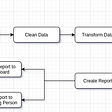## webserver setup on docker container using ansible-playbook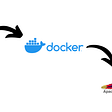## How we scaled Robinhood’s brokerage system for greater reliability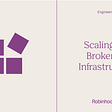## Deploy Django using UWSGI + Nginx in AWS Lightsail with Ubuntu 20.14 LTS## Lambda Bi-Weekly Report- Main Net Data Monitoring System Has Been Released## A working gitlab Laravel pipeline with PHPUnit, code style, and more## Introduction to MINIO file operation with Java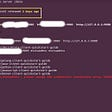## Fibonacci TDD Kata in Golang — from Zero to Hero in less than 5 minutes## A51F221B

I am a Cyber Security Engineer(currently studying) and a technology enthusiast.Looking forward to engage with the Medium community and share knowledge!

## Simple sHell: Explain ls -l *c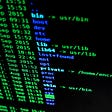## Basics of Assembly language : Part 3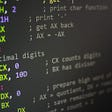## But what is the shell ?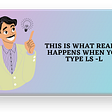## Farnell ships Arduino Nano and Raspberry Pi Pico development boards